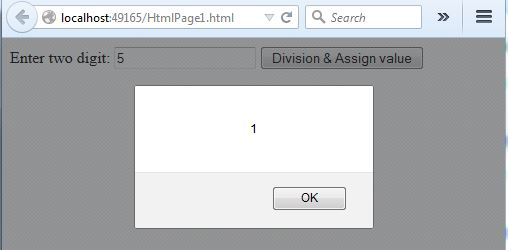#### JavaScript > Assignment Operators

##### How to divide and assign value in JavaScript?

To divide and assign value in JavaScript, we can follow this approach.

```   <script>
function Divisionassignvalue() {
var a = document.getElementById("txtA").value;
var result = 5;
result /= parseInt(a);  //* result = result / 5;
}
</script>

Enter two digit:
<input type="text" id="txtA" name="txtA" />
<input type="button" name="btnDivisionassignvalue" value="Division & Assign value"
onclick="Divisionassignvalue()" />
```

In the above code snippet, we have one textbox. On click of button, we are calling the `Divisionassignvalue`function that divide and assign the number and show result in the alert. Same as previous post, the value of text box is being parsed into integer and being divided by 5.

OUTPUTViews: 2655 | Post Order: 22